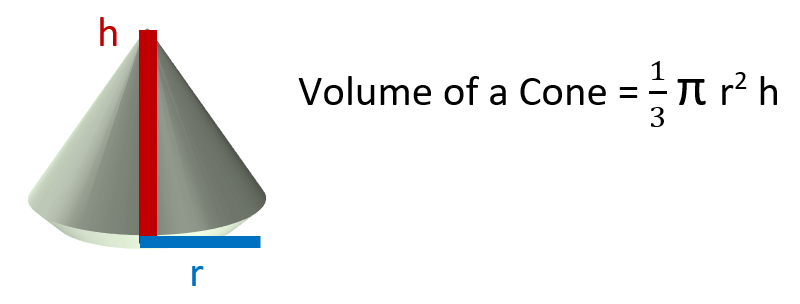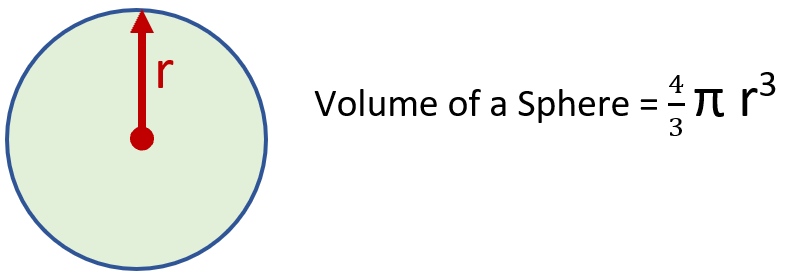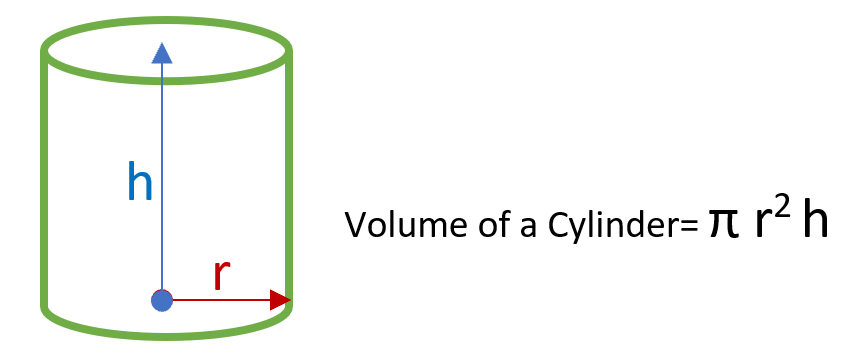# Math Worksheets Land

Math Worksheets For All Ages

# Math Worksheets Land

Math Worksheets For All Ages

# Volumes of Cones, Cylinders, and Spheres Worksheets

How do you find the volume of cones, cylinders, and spheres? There are many ways to describe an object, from their color, their shape and their size. Apart from these ways, we describe objects with their volumes too. In math, volume is defined as the three-dimensional space occupied by an object. Three-dimensional objects/ shapes are those solid figures that possess three dimensions, i.e., height, length, and width. We all are familiar with the shape of an ice-cream cone, a tennis ball, and food can. Below, we have discussed how to figure out the volume of these shapes. Volume of a Cone - Ice-cream cones, or mathematically put, cones are 3D figures with two faces and one edge. Simply put, cones are pointy at one end and flat on the other end. That means they have a point on one end and a circle on the other end. To find the volume of a cone, we use the following formula: V= 1/3 Π x r2 x h Where, Π (constant) = 3.14 r is the radius of a circle. h is the height of the cone. Volume of a Sphere - Tennis balls or basketballs are one of the few examples from our daily life of spherical shape. However, in math, spheres are defined as a perfectly symmetrical 3D shape with zero vertices and one surface. Each point on the surface of the sphere is at the same distance from its center. To find the volume of the sphere, we use the following formula. V= 4/3 x Π x r3 Where, Π (constant) is 3.14. r is the radius of the sphere. Volume of a Cylinder - We all enjoy a chilled soft drink can on a warm summer day. The shape of these cans is termed as cylindrical. Cylinders have two round shapes at both ends and two parallel lines joining the round ends. To find the formula of a cylinder, we use the formula. V = Π x r2 x h. Where, Π (constant) is taken as 3.14. r is the radius of the circular end of the cylinder. h is the height of the cylinder. This selection of lessons and worksheets helps students learn to calculate the volume of three critical three-dimensional shapes.

### Aligned Standard: Grade 8 Geometry - 8.G.C.9

• Cylinder Volume Step-by-Step Lesson- We work you through finding the volume of a cylinder. You can think of it as a Coke can. You will need to remember to square the radius.
• Guided Lesson - We find the volume of everyday random objects. It's amazing how everything comes back to simple shapes.
• Guided Lesson Explanation - I try to enlighten you on the use of premade formulas that are used to determine the measures found here. We show you how to determine which variable goes where.
• Independent Practice - It helps a great deal to draw pictures of these scenarios. These are pretty extreme word problems.
• Matching Worksheet - I used matching units in this set. I normally don't but they really don't help you answer the problems.
• Answer Keys - These are for all the unlocked materials above.

### Homework Sheets

I formed all the questions as word problems.

• Homework 1 - Since we need to find the final answer in cubic centimeters all measures first need to be in centimeters.
• Homework 2 - Maria has a hat that is cone shaped. The hat is 9 cm long and has a 4 cm opening at the bottom. Find the volume of the hat. Ask yourself what the shape of a hat is.
• Homework 3 - A capsule of medicine is in the shape of a sphere of diameter 6 mm. How much medicine is needed to fill this capsule? This is a real life problem for you to ponder.

### Practice Worksheets

Don't forgot to properly label the units. Sometimes students forget that units are 50% of the answer.

• Practice 1 - A glass has a diameter of 8 cm and a height of 14 cm. What volume of water will it take to fill in the glass?
• Practice 2 - A test-tube is in shape of a cylinder. It has a radius of 2 cm and height of 7 cm. What is the volume of the test-tube?
• Practice 3 - A spinning top is in the shape of a cone has a diameter of 2 inch and its height is 3 inch. Find the volume of the spinning top.

### Math Skill Quizzes

When I created the problems I created a list of real life things that were in the three shapes and went from there.

• Quiz 1 - In a conical tank, the depth of water is 1.5 yards and radius of the water level is 0.5 yards. Find the volume of water.
• Quiz 2 - The diameter of the base of a cone is 6 m and its height is 20 m. Find the volume of the cone.
• Quiz 3 - The diameter of the base of a cone is 10 cm and its height is 16 cm. Find the volume of the cone.

### Quick Review on The Equations Used with Calculating These Measures

Working with Cones - We really only need to determine two measures of our cone in order to be able to determine the volume. The first measure is the height as you can see in the red line below. This is the measure from the base of the cone at a right angle to the exact upper most center point of the cone. The other measure (radius) is signified by the blue line below. That is the measure from the center of the base of the cone to the outer edge. You may remember that radius is half of the diameter. Once you have those two measures, it is pretty simple using the provided equation. Start by squaring the value of the radius. From there multiply that value by height and then Pi (3.14). Once you find those products, just divide it by 3.Working with Spheres - Sphere only require you to determine one measure to solve them. You need to find the measure of the radius. This is the measure from the direct center of the sphere to edge of the sphere in any direction. Once you have that value, you cube it. Take that value and multiply it by Pi (3.14) and 4/3.Working with Cylinders - Cylinders require two measures. The first measure you can calculate from either the circular base or top of the geometric shape, since it is a right-angle cylinder, they will be the same. This is much like the measure in the cylinder, but you can take your choice of where to measure from. The last measure is the height, once again, just like the cylinder. You will then square the measure of the radius and find the product of that measure with both height and pi (3.14). Unlike the others, there is no need to worry about a fraction here.Unlock all the answers, worksheets, homework, tests and more!
Save Tons of Time! Make My Life Easier Now

## Thanks and Don't Forget To Tell Your Friends!

I would appreciate everyone letting me know if you find any errors. I'm getting a little older these days and my eyes are going. Please contact me, to let me know. I'll fix it ASAP.